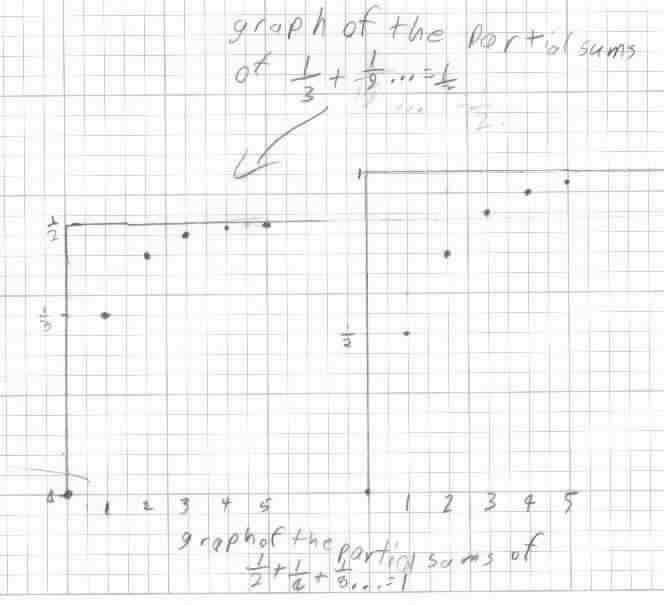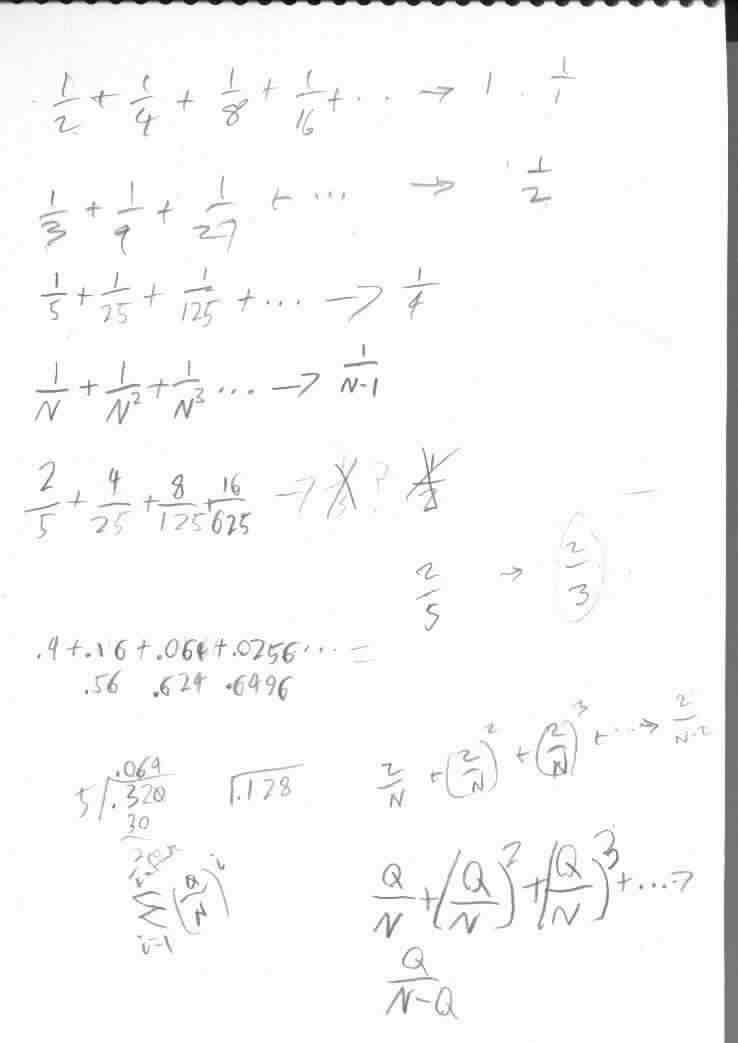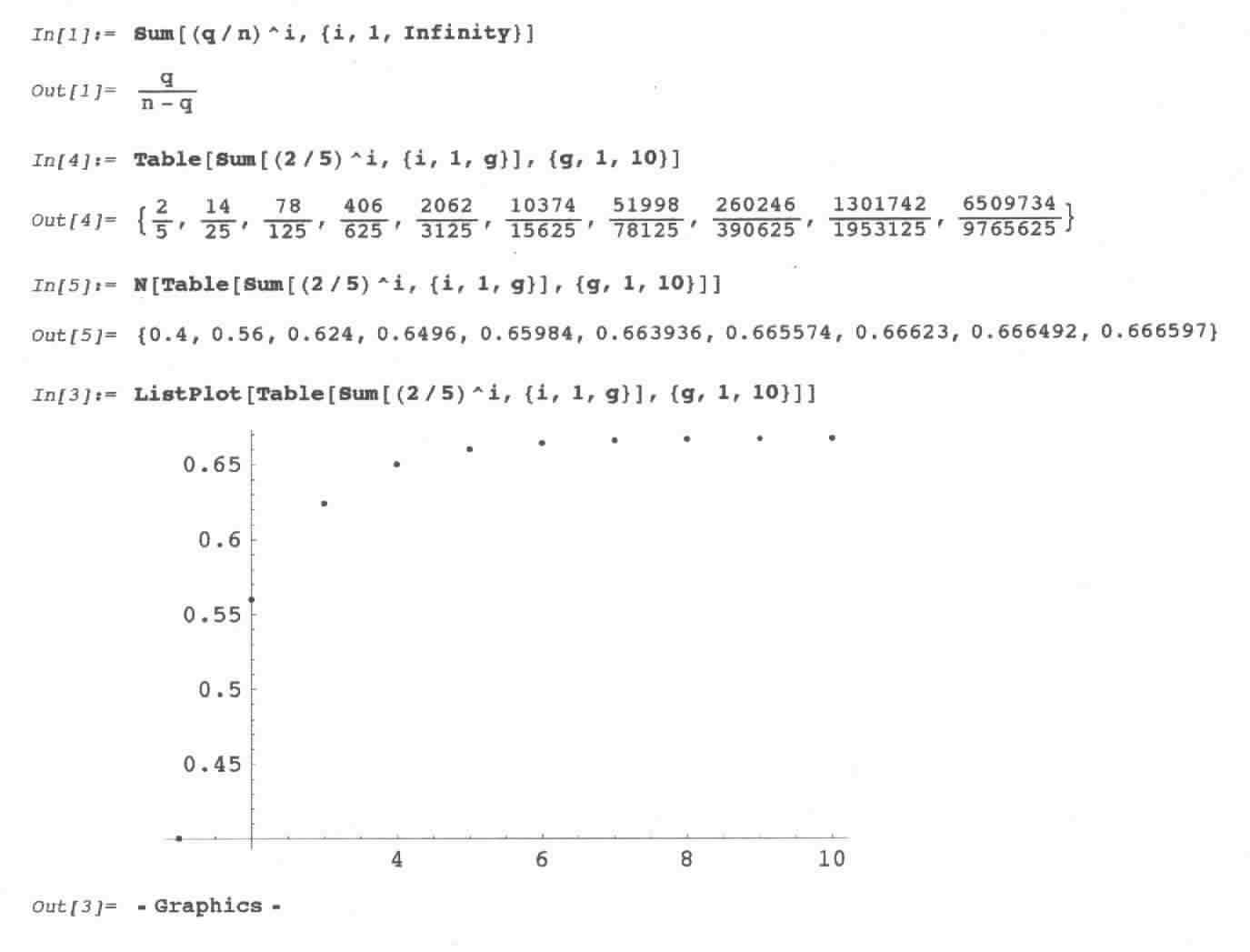Geoffrey, as a 5th grader,  generalizes Q/N + (Q/N)2 + (Q/N)3 + (Q/N)4 + ...Geoffrey found patterns in the numerators and denominators of the partials sums. Then he found that if the infinite series started with 1/N it would go to 1/(N-1) below.That night his Dad worked with Geoffrey using Mathematica to do the infinite sum, shown below:To Geoffrey's work on powers of powers
To Geoffrey's work on the area under curves and the integral
To Geoffrey's work on the Fibonacci numbers
To Geoffrey's work on graphs
To Geoffrey's work on the 6 trig functions
To other discoveries
To order Don's materials
Mathman home